### Bugzzy- Part 2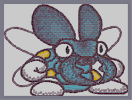Hover over the thumbnail for a full-size version.

Author LordOfPeanuts author:lordofpeanuts bitesized n-art nonplayable rated 2007-12-04 2007-12-04 4 by 35 people. \$Bugzzy- Part 2#LordOfPeanuts#none#00000000000000000000000000000000000000000000000000000000000000000000000000000000000000000000000000000000000000000000000000000000000000000000000000000000000000000000000000000000000000000000000000000000000000000000000000000000000000000000000000000000000000000000000000000000000000000000000000000000000000000000000000000000000000000000000000000000000000000000000000000000000000000000000000000000000000000000000000000000000000000000000000000000000000000000000000000000000000000000000000000000000000000000000000000000000000000000000000000000000000000000000000000000000000000000000000000000000000000000000000000000000000000000000000000000000000000000000000000000000000000000000000000000000000000000000000000000000000000|5^636,416!5^630,479!5^382,383!5^386,456!5^416,480!5^484,501!5^516,506!5^443,497!5^398,301!5^404,287!5^607,282!5^604,290!5^604,303!5^609,304!5^605,294!5^613,295!5^407,308!5^400,302!5^406,311!0^264,354!0^264,348!0^270,342!0^276,342!0^282,342!0^288,342!0^288,336!0^294,336!0^294,330!0^294,324!0^294,318!0^294,312!0^288,312!0^288,306!0^282,306!0^276,306!0^270,306!0^264,306!0^258,312!0^252,312!0^246,312!0^240,318!0^234,318!0^228,324!0^222,330!0^216,336!0^222,336!0^228,330!0^234,324!0^240,324!0^246,318!0^252,318!0^258,318!0^264,312!0^276,312!0^270,312!0^282,312!0^258,348!0^264,342!0^270,336!0^276,336!0^282,336!0^288,330!0^282,330!0^234,336!0^240,330!0^246,324!0^240,342!0^246,336!0^252,330!0^258,324!0^264,318!0^270,324!0^276,318!0^288,318!0^288,324!0^282,324!0^276,330!0^264,330!0^258,336!0^252,342!0^324,462!0^324,456!0^324,450!0^330,438!0^330,444!0^324,432!0^288,426!0^318,426!0^294,426!0^312,426!0^288,432!0^300,420!0^306,420!0^312,420!0^300,426!0^282,456!0^288,450!0^288,444!0^288,438!0^294,432!0^306,426!0^288,456!0^294,438!0^324,444!0^324,438!0^318,432!0^318,444!0^318,438!0^318,450!0^318,456!0^300,438!0^306,432!0^312,438!0^306,444!0^294,444!0^300,450!0^294,456!0^306,456!0^312,450!0^558,426!0^552,426!0^564,426!0^570,432!0^576,432!0^582,438!0^588,444!0^594,450!0^600,456!0^600,462!0^600,468!0^594,474!0^588,474!0^576,480!0^570,480!0^564,474!0^552,432!0^558,432!0^564,432!0^564,468!0^570,474!0^576,474!0^588,468!0^594,468!0^594,462!0^594,456!0^588,450!0^582,444!0^576,438!0^570,438!0^564,462!0^570,468!0^558,438!0^558,450!0^564,444!0^576,444!0^570,450!0^564,456!0^576,456!0^582,450!0^588,456!0^582,462!0^576,468!0^570,462!0^636,336!0^642,342!0^636,342!10^222,408!10^222,414!10^222,420!10^210,426!10^216,426!10^222,426!10^228,426!10^228,420!10^228,432!10^234,432!10^240,432!10^246,432!10^246,438!10^252,432!10^258,432!10^264,432!10^264,426!10^270,426!10^276,426!10^276,420!10^276,414!10^282,414!10^282,420!10^282,426!10^282,432!10^282,438!10^282,444!10^282,450!10^282,408!10^288,408!10^288,402!10^282,402!10^276,438!10^276,444!10^276,450!10^276,456!10^270,456!10^276,396!10^282,396!10^276,390!10^282,390!10^270,384!10^276,384!10^282,384!10^264,378!10^270,378!10^276,378!10^282,378!10^294,372!10^252,372!10^288,372!10^282,372!10^276,372!10^270,372!10^264,372!10^258,372!10^240,366!10^246,366!10^252,366!10^270,366!10^312,366!10^276,366!10^288,366!10^294,366!10^300,366!10^306,366!10^192,360!10^234,360!10^228,360!10^222,360!10^216,360!10^210,360!10^204,360!10^198,360!10^264,360!10^258,354!10^252,354!10^252,348!10^240,348!10^246,348!10^234,342!10^210,342!10^228,342!10^222,342!10^216,342!10^204,342!10^198,348!10^192,348!10^180,354!10^186,354!10^174,360!10^180,360!10^192,366!10^168,366!10^174,366!10^180,366!10^186,366!10^174,372!10^162,372!10^168,372!10^162,378!10^156,378!10^150,384!10^144,390!10^144,396!10^138,396!10^138,402!10^132,408!10^132,414!10^132,420!10^132,426!10^132,432!10^132,438!10^132,444!10^132,450!10^138,450!10^138,456!10^138,462!10^144,462!10^144,468!10^150,468!10^150,474!10^156,474!10^162,474!10^162,480!10^174,480!10^168,480!10^174,486!10^240,486!10^180,486!10^186,486!10^192,486!10^198,486!10^204,486!10^210,486!10^216,486!10^222,486!10^228,486!10^234,486!10^204,492!10^192,492!10^198,492!10^228,480!10^246,480!10^240,480!10^234,480!10^246,474!10^252,474!10^252,468!10^264,468!10^258,468!10^264,462!10^270,462!10^318,462!10^276,462!10^282,462!10^288,462!10^294,462!10^300,462!10^306,462!10^312,462!10^186,534!10^186,558!10^186,540!10^186,546!10^186,552!10^192,564!10^198,570!10^204,570!10^210,570!10^216,576!10^222,576!10^228,576!10^234,576!10^240,576!10^246,576!10^252,576!10^258,576!10^264,576!10^354,576!10^360,576!10^348,576!10^342,576!10^336,576!10^330,576!10^366,570!10^372,570!10^378,564!10^378,558!10^384,552!10^384,546!10^384,540!10^384,534!10^378,534!10^378,528!10^378,522!10^372,522!10^372,516!10^366,516!10^366,510!10^192,528!10^192,522!10^198,516!10^204,510!10^210,510!10^210,504!10^216,504!10^222,498!10^228,498!10^234,498!10^234,492!10^240,492!10^246,492!10^252,492!10^252,486!10^258,486!10^264,486!10^270,486!10^306,486!10^276,486!10^300,486!10^288,486!10^282,486!10^294,486!10^312,492!10^336,492!10^330,492!10^324,492!10^318,492!10^336,498!10^348,498!10^342,498!10^360,510!10^366,504!10^348,504!10^360,504!10^354,504!10^324,576!10^318,576!10^306,576!10^270,576!10^288,576!10^288,576!10^300,576!10^282,576!10^294,576!10^312,576!10^276,576!10^318,468!10^342,468!10^324,468!10^330,468!10^336,468!10^336,462!10^336,456!10^336,450!10^342,474!10^348,474!10^354,474!10^354,480!10^360,480!10^360,498!10^360,492!10^360,486!10^384,498!10^378,498!10^372,498!10^366,498!10^498,504!10^378,504!10^492,504!10^486,504!10^480,504!10^474,504!10^468,504!10^462,504!10^450,504!10^456,504!10^444,504!10^438,504!10^432,504!10^426,504!10^420,504!10^414,504!10^408,504!10^402,504!10^396,504!10^384,504!10^390,504!10^480,510!10^570,510!10^486,510!10^492,510!10^498,510!10^504,510!10^510,510!10^516,510!10^522,510!10^528,510!10^534,510!10^540,510!10^546,510!10^552,510!10^558,510!10^564,510!10^342,444!10^348,444!10^540,516!10^546,516!10^540,522!10^534,522!10^534,528!10^528,528!10^528,534!10^534,534!10^522,534!10^516,534!10^510,540!10^504,540!10^498,540!10^492,546!10^486,546!10^480,546!10^474,546!10^468,546!10^462,546!10^462,552!10^456,552!10^450,552!10^420,552!10^426,552!10^432,552!10^438,552!10^444,552!10^456,546!10^450,546!10^390,546!10^396,546!10^402,546!10^408,546!10^414,546!10^426,546!10^420,546!10^432,546!10^594,504!10^546,504!10^588,504!10^582,504!10^576,504!10^570,504!10^564,504!10^558,504!10^552,504!10^534,540!10^540,546!10^552,552!10^546,552!10^558,552!10^564,558!10^744,558!10^570,558!10^576,558!10^582,558!10^588,558!10^594,558!10^600,558!10^606,558!10^612,558!10^618,558!10^624,558!10^630,558!10^636,558!10^642,558!10^648,558!10^654,558!10^660,558!10^666,558!10^672,558!10^678,558!10^684,558!10^690,558!10^696,558!10^702,558!10^708,558!10^714,558!10^720,558!10^726,558!10^732,558!10^738,558!10^744,552!10^750,552!10^756,552!10^762,546!10^768,546!10^768,540!10^768,534!10^768,528!10^768,522!10^762,522!10^762,516!10^756,516!10^750,510!10^738,504!10^744,504!10^732,498!10^726,498!10^708,492!10^714,492!10^720,492!10^690,486!10^708,486!10^696,486!10^702,486!10^690,480!10^684,480!10^678,480!10^672,480!10^312,360!10^318,360!10^324,354!10^324,348!10^330,348!10^276,300!10^282,294!10^294,294!10^288,294!10^330,294!10^300,294!10^306,294!10^312,294!10^318,294!10^324,294!10^324,300!10^330,300!10^330,306!10^330,312!10^330,324!10^330,318!10^336,324!10^336,330!10^330,342!10^336,342!10^336,336!10^336,330!10^342,330!10^342,336!10^342,342!10^612,462!10^606,456!10^636,348!10^666,348!10^642,348!10^648,348!10^654,348!10^660,348!10^642,354!10^684,354!10^648,354!10^654,354!10^660,354!10^666,354!10^672,354!10^678,354!10^690,360!10^696,360!10^702,360!10^708,366!10^714,366!10^726,372!10^720,372!10^726,378!10^732,384!10^738,390!10^738,396!10^738,432!10^738,426!10^738,420!10^738,414!10^738,408!10^738,402!10^744,414!10^744,408!10^744,402!10^732,432!10^732,438!10^726,444!10^720,450!10^714,456!10^708,456!10^702,462!10^678,462!10^684,462!10^690,462!10^696,462!10^672,468!10^678,468!10^684,468!10^666,474!10^672,474!10^660,480!10^654,480!10^648,486!10^642,486!10^636,486!10^636,492!10^630,492!10^624,492!10^618,492!10^618,498!10^612,498!10^606,498!10^600,498!10^684,456!10^690,456!10^690,450!10^696,450!10^696,408!10^696,414!10^696,420!10^696,426!10^696,432!10^696,438!10^696,444!10^690,402!10^690,396!10^684,390!10^684,384!10^678,384!10^678,378!10^672,378!10^672,372!10^666,372!10^666,366!10^660,366!10^660,360!10^654,360!10^378,372!10^342,372!10^372,372!10^366,372!10^360,372!10^354,372!10^348,372!10^342,378!10^336,378!10^336,384!10^330,384!10^330,420!10^330,390!10^330,396!10^330,402!10^330,408!10^330,414!10^324,402!10^324,408!10^324,396!10^336,420!10^336,426!10^342,426!10^342,432!10^348,438!10^348,432!10^354,438!10^354,402!10^354,432!10^354,426!10^354,420!10^354,414!10^354,408!10^360,444!10^360,390!10^360,438!10^360,432!10^360,426!10^360,420!10^360,408!10^360,402!10^360,396!10^366,390!10^366,384!10^366,378!10^372,378!10^366,444!10^366,450!10^366,456!10^372,456!10^372,462!10^378,462!10^378,468!10^384,468!10^384,474!10^390,474!10^390,480!10^396,480!10^396,486!10^402,486!10^402,480!10^408,492!10^408,486!10^414,486!10^414,492!10^420,492!10^426,492!10^420,498!10^450,498!10^426,498!10^432,498!10^438,498!10^444,498!10^348,348!10^354,354!10^360,360!10^360,366!10^384,366!10^366,366!10^372,366!10^378,366!10^366,360!10^360,354!10^390,360!10^384,360!10^390,354!10^396,354!10^402,354!10^348,342!10^354,342!10^360,342!10^360,348!10^414,348!10^372,348!10^366,348!10^378,348!10^384,348!10^390,348!10^396,348!10^402,348!10^408,348!10^318,288!10^318,282!10^318,276!10^318,270!10^408,342!10^414,342!10^420,342!10^426,342!10^432,342!10^420,336!10^474,336!10^426,336!10^432,336!10^438,336!10^444,336!10^450,336!10^456,336!10^462,336!10^468,336!10^432,330!10^426,330!10^432,324!10^438,324!10^432,318!10^438,318!10^444,318!10^450,318!10^450,324!10^450,330!10^456,324!10^456,330!10^462,330!10^438,288!10^438,294!10^438,300!10^438,306!10^438,312!10^432,288!10^432,282!10^432,276!10^426,276!10^426,270!10^420,270!10^420,264!10^414,264!10^408,264!10^408,258!10^402,258!10^390,258!10^396,258!10^378,258!10^372,258!10^384,258!10^366,258!10^360,258!10^360,264!10^354,264!10^348,270!10^336,282!10^330,288!10^330,282!10^330,276!10^342,276!10^336,276!10^342,270!10^336,270!10^330,270!10^324,270!10^336,264!10^348,264!10^342,264!10^342,258!10^348,258!10^354,258!10^354,252!10^348,252!10^354,246!10^360,246!10^366,246!10^366,240!10^372,240!10^378,240!10^360,240!10^582,498!10^576,498!10^576,492!10^558,492!10^570,492!10^564,492!10^558,456!10^558,462!10^558,468!10^558,474!10^558,480!10^558,486!10^552,486!10^552,438!10^552,480!10^552,474!10^552,468!10^552,462!10^552,450!10^552,456!10^552,444!10^546,444!10^546,378!10^546,438!10^546,426!10^546,432!10^546,420!10^546,414!10^546,408!10^546,402!10^546,396!10^546,390!10^546,384!10^546,486!10^540,486!10^540,480!10^534,480!10^528,480!10^522,480!10^522,474!10^516,474!10^510,474!10^504,468!10^498,468!10^498,462!10^492,462!10^486,462!10^486,456!10^480,456!10^474,450!10^480,450!10^480,444!10^486,444!10^486,438!10^492,438!10^498,438!10^504,438!10^510,438!10^516,438!10^522,438!10^540,438!10^540,414!10^540,432!10^540,426!10^540,420!10^534,426!10^534,432!10^528,432!10^522,432!10^516,432!10^498,432!10^492,432!10^504,426!10^492,420!10^498,420!10^498,426!10^492,414!10^486,414!10^480,414!10^474,414!10^474,408!10^282,300!10^288,300!10^294,306!10^300,312!10^300,318!10^300,324!10^300,330!10^300,336!10^294,342!10^276,348!10^270,354!10^270,348!10^600,450!10^594,444!10^564,486!10^570,486!10^576,486!10^582,486!10^588,480!10^594,480!10^600,474!10^606,468!10^588,438!10^582,432!10^576,426!10^570,426!10^564,420!10^558,420!10^552,420!10^648,342!10^642,336!10^636,330!10^336,438!10^336,444!10^330,432!10^324,426!10^318,420!10^288,420!10^294,420!10^300,414!10^306,414!10^312,414!10^270,300!10^264,300!10^258,306!10^252,306!10^246,306!10^240,312!10^234,312!10^228,318!10^222,324!10^216,330!10^210,336!10^330,462!10^330,456!10^330,450!10^468,408!10^462,408!10^462,402!10^456,402!10^456,396!10^456,372!10^456,390!10^456,384!10^456,378!10^450,384!10^450,390!10^450,396!10^462,384!10^462,360!10^462,366!10^462,372!10^462,378!10^486,384!10^468,384!10^474,384!10^480,384!10^480,378!10^474,372!10^474,366!10^474,360!10^468,360!10^468,354!10^474,354!10^474,348!10^480,348!10^468,342!10^570,342!10^474,342!10^480,342!10^486,342!10^492,342!10^498,342!10^504,342!10^510,342!10^516,342!10^522,342!10^528,342!10^534,342!10^540,342!10^546,342!10^552,342!10^558,342!10^564,342!10^492,390!10^498,390!10^504,390!10^510,390!10^510,384!10^558,384!10^540,390!10^552,384!10^540,384!10^516,384!10^522,384!10^528,384!10^534,384!10^504,378!10^498,372!10^498,366!10^504,366!10^504,360!10^504,354!10^504,348!10^540,348!10^540,354!10^540,360!10^510,360!10^516,360!10^522,360!10^528,360!10^534,360!10^534,354!10^534,348!10^528,348!10^516,348!10^522,348!10^510,348!10^510,354!10^516,354!10^522,354!10^528,354!10^546,360!10^546,366!10^552,366!10^552,372!10^564,378!10^570,372!10^570,348!10^570,354!10^570,360!10^570,366!10^564,348!10^576,348!10^576,366!10^576,354!10^576,360!10^582,360!10^582,354!10^588,354!10^588,360!10^594,360!10^594,366!10^600,366!10^600,372!10^606,372!10^606,378!10^612,378!10^618,390!10^612,402!10^612,396!10^612,384!10^612,390!10^606,402!10^606,408!10^600,408!10^594,414!10^600,414!10^600,420!10^606,420!10^612,426!10^618,426!10^624,432!10^630,438!10^630,444!10^624,444!10^624,450!10^624,456!10^624,462!10^618,462!10^618,468!10^618,474!10^612,474!10^612,480!10^606,486!10^606,492!10^600,492!10^630,342!0^630,336!10^624,342!10^618,342!10^624,336!10^624,330!10^630,330!10^600,336!10^618,336!10^612,336!10^606,336!10^600,330!10^558,336!10^564,336!10^564,330!10^594,330!10^588,330!10^582,330!10^576,330!10^570,330!10^630,324!10^636,318!10^636,312!10^636,306!10^642,306!10^642,300!10^642,294!10^642,288!10^636,282!10^636,276!10^630,270!10^630,264!10^624,264!10^618,258!10^612,252!10^606,252!10^600,252!10^594,252!10^588,252!10^582,252!10^582,258!10^588,258!10^582,276!10^576,276!10^576,282!10^576,288!10^576,294!10^576,300!10^576,306!10^576,312!10^576,318!10^570,324!10^576,324!10^582,324!10^582,270!10^582,264!10^582,282!10^582,318!10^582,312!10^582,306!10^582,288!10^582,294!10^582,300!10^372,234!10^450,234!10^378,234!10^384,234!10^390,234!10^396,234!10^402,234!10^408,234!10^414,234!10^420,234!10^426,234!10^432,234!10^426,234!10^438,234!10^444,234!10^432,240!10^438,240!10^444,240!10^450,240!10^450,246!10^504,246!10^456,246!10^456,240!10^462,246!10^468,246!10^474,246!10^480,246!10^486,246!10^492,246!10^498,246!10^480,240!10^582,240!10^582,246!10^576,246!10^570,246!10^486,240!10^492,240!10^498,240!10^504,240!10^510,240!10^516,240!10^522,240!10^528,240!10^534,240!10^540,240!10^546,240!10^552,240!10^558,240!10^564,240!10^570,240!10^576,240!10^552,234!10^540,234!10^534,234!10^528,234!10^522,234!10^516,234!10^486,234!10^480,234!10^480,228!10^486,228!10^486,222!10^480,222!10^480,54!10^480,216!10^480,210!10^480,204!10^480,198!10^480,192!10^480,186!10^480,180!10^480,174!10^480,168!10^480,162!10^480,156!10^480,150!10^480,144!10^480,138!10^480,132!10^480,126!10^480,120!10^480,114!10^480,108!10^480,102!10^480,96!10^480,90!10^480,84!10^480,78!10^480,72!10^480,66!10^480,54!10^480,60!10^474,78!10^474,192!10^474,84!10^474,90!10^474,96!10^474,102!10^474,108!10^474,114!10^474,120!10^474,126!10^474,132!10^474,138!10^474,144!10^474,150!10^474,156!10^474,162!10^474,168!10^474,174!10^474,180!10^474,186!10^582,234!10^576,234!10^582,228!10^588,228!10^588,222!10^588,216!10^594,216!10^594,210!10^594,204!10^594,198!10^600,204!10^600,108!10^600,198!10^600,186!10^600,192!10^600,180!10^600,174!10^600,168!10^600,162!10^600,156!10^600,150!10^600,144!10^600,138!10^600,132!10^600,126!10^600,120!10^600,114!10^594,114!10^594,108!10^594,102!10^594,96!10^588,102!10^588,96!10^588,90!10^606,132!10^606,180!10^606,138!10^606,144!10^606,150!10^606,156!10^606,162!10^606,168!10^606,174!10^582,90!10^582,84!10^582,78!10^576,78!10^576,72!10^570,72!10^570,66!10^570,60!10^564,66!10^564,60!10^564,54!10^558,54!10^552,54!10^552,48!10^546,48!10^546,42!10^540,42!10^540,36!10^534,36!10^528,36!10^522,36!10^528,30!10^522,30!10^516,30!10^510,30!10^504,30!10^498,30!10^504,36!10^498,36!10^492,36!10^492,42!10^486,42!10^486,48!10^486,54!10^486,60!10^444,228!10^450,228!10^450,222!10^450,216!10^444,216!10^444,210!10^450,210!10^450,204!10^444,204!10^444,198!10^444,192!10^444,186!10^438,192!10^438,186!10^438,180!10^438,174!10^432,180!10^432,174!10^432,168!10^432,162!10^312,270!10^306,270!10^300,270!10^306,264!10^300,264!10^294,264!10^288,264!10^288,258!10^282,258!10^276,258!10^276,252!10^270,252!10^264,252!10^264,246!10^258,246!10^258,240!10^252,240!10^246,240!10^252,234!10^246,234!10^426,168!10^426,162!10^426,156!10^426,150!10^420,156!10^420,150!10^420,144!10^414,144!10^414,138!10^414,132!10^408,138!10^408,132!10^408,126!10^402,126!10^402,120!10^396,120!10^396,114!10^390,114!10^390,108!10^384,108!10^390,102!10^384,102!10^378,102!10^372,96!10^378,96!10^384,96!10^378,90!10^372,90!10^366,90!10^360,84!10^366,84!10^372,84!10^360,78!10^354,78!10^354,72!10^348,72!10^342,72!10^336,66!10^342,66!10^348,66!10^336,60!10^330,60!10^324,60!10^330,54!10^324,54!10^318,54!10^312,54!10^318,48!10^312,48!10^306,48!10^300,48!10^300,42!10^294,42!10^288,42!10^282,42!10^288,36!10^282,36!10^276,36!10^270,36!10^264,36!10^258,36!10^252,36!10^234,36!10^240,36!10^246,36!10^252,30!10^258,30!10^264,30!10^240,42!10^234,42!10^228,42!10^228,48!10^222,48!10^222,54!10^222,60!10^216,60!10^216,54!10^216,66!10^210,66!10^210,72!10^210,78!10^204,78!10^204,108!10^204,84!10^204,90!10^204,96!10^204,102!10^198,90!10^198,174!10^198,96!10^198,108!10^198,102!10^198,114!10^198,120!10^198,126!10^198,132!10^198,138!10^198,144!10^198,150!10^198,156!10^198,162!10^198,168!10^192,144!10^192,138!10^192,132!10^192,126!10^204,168!10^204,192!10^204,174!10^204,180!10^204,186!10^210,186!10^210,204!10^210,198!10^210,192!10^216,198!10^216,204!10^216,210!10^222,210!10^222,216!10^228,216!10^228,222!10^234,222!10^234,228!10^240,228!3^144,420!3^150,438!3^162,450!3^180,462!3^204,468!3^228,462!3^252,456!3^246,462!3^234,468!3^222,474!3^210,474!3^198,474!3^192,474!3^180,468!3^168,468!3^162,456!3^150,450!3^144,444!3^144,432!3^264,450!3^240,414!3^252,408!3^264,396!3^264,408!3^252,420!3^258,414!3^204,540!3^216,552!3^246,552!3^276,558!3^318,552!3^348,552!3^366,540!3^204,552!3^216,558!3^228,564!3^234,558!3^246,558!3^258,564!3^264,564!3^282,564!3^294,558!3^306,558!3^300,564!3^318,564!3^330,558!3^342,564!3^366,552!3^360,558!3^354,564!3^354,486!3^348,492!3^264,480!3^204,348!3^714,384!3^720,402!3^714,426!3^708,444!3^720,432!3^714,438!3^726,420!3^726,408!3^726,396!3^720,390!10^405,281!10^409,301!10^409,306!10^405,310!10^404,311!10^406,283!10^408,312!10^608,309!10^602,302!10^602,296!10^602,290!10^605,282!10^607,276!10^611,299!10^613,299!10^611,303!10^607,304!10^606,301!3^552,528!3^576,534!3^594,534!3^624,540!3^648,534!3^678,540!3^708,540!3^738,522!3^726,534!3^744,528!3^750,534!3^744,540!3^738,534!3^732,540!3^726,546!3^714,546!3^702,546!3^696,540!3^684,540!3^672,546!3^684,546!3^666,540!3^654,540!3^636,540!3^654,546!3^690,534!3^558,540!3^546,534!3^564,540!3^576,546!3^588,540!3^606,546!3^600,546!3^612,540!3^624,546!3^636,546!3^744,516!3^756,534!3^591,495!10^399,295!10^399,303!10^403,314!10^403,308!10^401,305!10^401,301!10^401,297!10^401,289!10^403,286!10^605,312!0^270,342!0^276,342!0^282,342!10^270,348!10^300,342!10^300,330!10^300,318!0^294,318!0^294,324!0^294,330!10^300,294!10^294,294!10^300,312!10^294,306!10^294,300!10^318,294!10^324,294!10^330,294!10^330,300!10^330,306!10^330,318!10^336,324!10^336,330!10^330,342!10^330,348!10^324,354!10^288,348!10^282,348!8^570,228,0!8^564,228,0!8^558,228,0!8^552,228,0!8^546,228,0!8^540,228,0!8^534,228,0!8^528,228,0!8^522,228,0!8^516,228,0!8^510,228,0!8^504,228,0!8^498,228,0!8^492,228,0!8^486,228,0!10^486,222!10^486,228!10^486,234!10^480,234!10^480,228!10^480,222!10^492,240!10^498,240!10^504,240!10^510,240!10^516,240!10^522,240!10^528,240!10^534,240!10^540,240!10^516,234!10^522,234!10^528,234!10^534,234!10^540,234!10^546,234!10^552,240!10^558,240!10^564,240!10^570,240!10^576,240!10^552,234!10^576,234!8^576,210,0!8^570,210,0!8^564,210,0!8^558,210,0!8^552,210,0!8^546,210,0!8^540,210,0!8^534,210,0!8^528,210,0!8^522,210,0!8^516,210,0!8^510,210,0!8^504,210,0!8^498,210,0!8^492,210,0!8^486,210,0!10^480,216!10^480,210!10^480,204!8^582,192,0!8^576,192,0!8^570,192,0!8^564,192,0!8^558,192,0!8^552,192,0!8^540,192,0!8^534,192,0!8^528,192,0!8^522,192,0!8^516,192,0!8^510,192,0!8^504,192,0!8^498,192,0!8^492,192,0!8^486,192,0!10^480,198!10^480,192!10^480,186!8^582,174,0!8^576,174,0!8^570,174,0!8^564,174,0!8^558,174,0!8^552,174,0!8^546,174,0!8^540,174,0!8^534,174,0!8^528,174,0!8^522,174,0!8^510,174,0!8^504,174,0!8^498,174,0!8^492,174,0!8^486,174,0!10^480,180!10^480,174!10^480,168!8^582,156,0!8^576,156,0!8^570,156,0!8^564,156,0!8^558,156,0!8^552,156,0!8^546,156,0!8^540,156,0!8^528,156,0!8^522,156,0!8^516,156,0!8^510,156,0!8^504,156,0!8^498,156,0!8^492,156,0!8^486,156,0!10^480,162!10^480,156!10^480,150!8^480,210,0!8^480,192,0!8^480,174,0!8^480,156,0!10^480,216!10^480,204!10^480,210!10^474,198!10^474,192!10^474,186!10^474,180!10^474,174!10^474,168!10^474,162!10^474,156!10^474,150!10^480,150!10^480,156!10^480,162!10^480,168!10^480,174!10^480,180!10^480,186!10^480,192!10^480,198!10^480,204!8^582,138,0!8^576,138,0!8^570,138,0!8^564,138,0!8^558,138,0!8^552,138,0!8^546,138,0!8^540,138,0!8^534,138,0!8^528,138,0!8^522,138,0!8^516,138,0!8^510,138,0!8^504,138,0!8^498,138,0!8^492,138,0!8^486,138,0!8^480,138,0!10^480,138!10^480,132!10^474,132!10^474,138!10^474,144!10^480,144!8^582,120,0!8^576,120,0!8^570,120,0!8^564,120,0!8^558,120,0!8^552,120,0!8^546,120,0!8^540,120,0!8^534,120,0!8^528,120,0!8^522,120,0!8^516,120,0!8^510,120,0!8^504,120,0!8^498,120,0!8^492,120,0!8^486,120,0!8^480,120,0!10^480,120!10^480,114!10^474,114!10^474,120!10^480,126!8^576,102,0!10^588,102!10^588,96!8^570,102,0!8^564,102,0!8^558,102,0!8^552,102,0!8^546,102,0!8^540,102,0!8^534,102,0!8^528,102,0!8^522,102,0!8^516,102,0!8^510,102,0!8^504,102,0!8^498,102,0!8^492,102,0!8^486,102,0!8^480,102,0!10^480,102!10^480,96!10^474,96!10^474,102!10^474,108!10^480,108!8^564,84,0!8^558,84,0!8^552,84,0!8^546,84,0!8^540,84,0!8^534,84,0!8^528,84,0!8^522,84,0!8^516,84,0!8^510,84,0!8^504,84,0!8^498,84,0!8^492,84,0!8^486,84,0!8^480,84,0!10^480,84!10^480,78!10^474,78!10^474,84!10^474,90!10^480,90!8^552,66,0!10^564,66!10^564,60!10^558,60!10^576,78!8^546,66,0!8^540,66,0!8^534,66,0!8^528,66,0!8^522,66,0!8^516,66,0!8^510,66,0!8^504,66,0!8^498,66,0!8^492,66,0!8^486,66,0!8^480,66,0!10^480,72!10^480,66!10^480,54!10^480,60!10^486,60!8^534,48,0!10^546,48!10^546,42!10^540,42!8^528,48,0!8^522,48,0!8^516,48,0!8^510,48,0!8^504,48,0!8^498,48,0!8^492,48,0!10^486,48!10^486,54!10^486,42!10^492,42!10^534,36!10^528,36!10^522,36!8^486,48,0!10^486,54!10^486,48!10^486,42!10^492,42!10^492,48!10^413,300!10^607,276!10^603,284!10^608,278!10^613,295!10^607,292!10^405,296!10^405,320!10^404,317!10^403,315!10^400,309!10^400,305!10^400,301!10^400,298!10^400,295!10^403,287!10^406,285!10^412,301!10^412,304!10^412,308!10^410,312!10^408,315!10^407,317!10^609,278!10^607,282!10^604,284!10^603,289!10^603,295!10^603,305!10^606,310!10^613,296!10^613,299!10^612,304!10^609,308!3^516,60!3^492,84!3^516,84!3^492,60!3^540,60!3^540,84!3^564,84!3^564,108!3^540,108!3^516,108!3^492,108!3^492,132!3^516,132!3^540,132!3^564,132!3^588,132!3^588,156!3^588,180!3^564,180!3^564,156!3^516,156!3^492,156!3^492,180!3^492,204!3^516,204!3^516,180!3^540,180!3^540,204!3^564,204!3^588,204!3^564,228!3^540,228!3^516,228!3^492,228!3^504,48!3^528,48!3^504,72!3^528,72!3^552,72!3^552,96!3^576,120!3^576,96!3^504,96!3^528,96!3^504,120!3^504,144!3^504,168!3^504,192!3^504,216!3^528,216!3^552,216!3^552,120!3^576,144!3^576,168!3^576,192!3^576,216!12^516,96!12^504,84!12^492,96!12^504,108!12^492,120!12^504,132!12^492,144!12^504,156!12^492,168!12^504,180!12^492,192!12^504,204!12^492,216!12^516,216!12^504,228!12^540,192!12^528,204!12^528,108!12^528,84!12^516,72!12^492,72!12^504,60!12^516,48!12^528,60!12^540,72!12^552,60!12^552,84!12^540,96!12^540,120!12^552,108!12^564,96!12^576,108!12^588,120!12^564,120!12^588,144!12^576,156!12^588,168!12^576,180!12^588,192!12^576,204!12^564,216!12^540,216!12^528,228!12^552,228!12^576,228!12^552,204!12^564,192!12^564,168!12^564,144!3^552,192!3^528,168!3^528,144!3^528,120!3^552,144!3^552,168!3^528,192!3^540,156!12^552,132!12^516,120!12^516,144!12^528,132!12^528,156!12^528,180!12^516,168!12^516,192!12^540,168!12^540,144!12^552,156!12^552,180!3^516,48!3^504,60!3^492,72!3^516,72!3^528,60!3^504,84!3^492,96!3^504,108!3^492,120!3^504,132!3^492,144!3^504,156!3^492,168!3^504,180!3^492,192!3^504,204!3^492,216!3^504,228!3^516,216!3^528,228!3^540,216!3^552,228!3^564,216!3^552,204!3^576,228!3^576,204!3^564,192!3^576,180!3^588,192!3^564,168!3^516,120!3^516,96!3^528,108!3^540,120!3^552,132!3^564,144!3^576,156!3^588,144!12^576,132!3^576,132!3^588,120!3^576,108!3^564,120!3^552,108!3^540,96!3^528,84!3^540,72!3^552,84!3^564,96!3^576,108!3^576,90!3^564,72!3^540,48!3^552,60!3^588,108!3^588,168!3^510,36!3^510,54!3^510,66!3^510,78!3^498,78!3^498,90!3^498,54!3^504,42!3^528,42!3^534,60!3^522,60!3^492,78!3^498,102!3^492,120!3^540,60!3^558,78!3^534,66!3^546,72!3^486,114!3^486,150!3^486,174!3^486,192!3^486,120!3^492,114!3^492,138!3^486,192!3^498,216!3^504,222!3^498,228!3^492,204!3^492,198!3^570,84!3^588,120!10^482,237!10^479,226!10^476,217!10^476,213!10^486,230!10^482,215!10^478,195!10^473,181!10^476,205!10^476,64!10^477,58!10^478,52!10^483,48!10^497,31!10^491,34!10^490,38!10^488,40!10^484,41!10^482,43!10^481,45!10^481,47!10^481,51!10^480,54!10^480,59!10^477,63!10^475,67!10^475,68!10^500,27!10^496,32!10^502,31!10^509,25!10^511,29!10^520,28!10^526,28!10^530,28!10^536,31!10^542,35!10^547,37!10^554,42!10^557,47!10^552,42!10^563,52!10^572,62!10^578,68!10^582,74!10^587,81!10^591,87!10^600,101!10^596,94!10^600,103!10^604,109!10^604,113!10^606,118!10^607,126!10^607,122!10^609,133!10^611,142!10^612,151!10^610,158!10^609,166!10^608,172!10^610,133!10^608,121!10^605,185!10^602,193!10^602,200!10^599,208!10^595,213!10^592,221!10^588,225!10^582,231!10^580,233!10^571,233!10^581,224!10^587,216!10^594,210!10^593,205!10^598,188!10^599,180!10^599,169!10^599,157!10^599,139!10^595,118!10^528,528!10^534,522!10^534,516!10^534,510!10^528,510!10^522,510!10^510,510!10^516,510!10^372,522!10^366,516!10^366,510!10^372,504!10^504,510!10^498,510!10^492,510!10^486,510!10^480,510!10^474,510!8^396,534,2!8^402,534,2!8^408,534,2!8^414,534,2!8^426,534,2!10^510,540!10^498,540!10^504,540!10^492,540!10^492,546!10^486,546!10^516,534!10^498,540!10^492,546!10^486,546!10^480,546!10^474,546!10^468,546!10^456,546!10^450,546!10^462,546!10^432,546!10^426,546!10^420,546!10^408,546!10^402,546!10^396,546!10^390,546!10^384,534!8^384,516,2!8^396,516,2!8^402,516,2!8^408,516,2!8^414,516,2!8^420,516,2!8^426,516,2!8^432,516,2!10^378,522!10^372,504!8^450,516,2!8^462,516,2!8^468,516,2!10^474,510!8^480,516,2!8^486,516,2!8^492,516,2!8^498,516,2!8^504,516,2!8^510,516,2!8^516,516,2!8^522,516,2!8^528,516,2!10^534,516!10^534,522!8^534,516,2!10^534,522!10^540,522!10^540,516!10^534,516!10^540,510!10^534,510!10^546,516!10^480,510!10^486,510!10^492,510!10^474,510!10^498,510!10^504,510!10^510,510!10^516,510!10^522,510!10^528,510!10^378,504!10^396,504!10^390,504!10^408,504!10^408,504!10^420,504!10^438,504!10^444,504!10^456,504!10^438,504!10^426,504!10^426,504!10^450,504!10^462,504!3^378,516!3^384,528!3^396,540!3^420,540!3^528,516!3^408,540!8^432,534,2!8^444,534,2!8^450,534,2!8^456,534,2!8^462,534,2!8^468,534,2!8^474,534,2!8^480,534,2!8^486,534,2!8^492,534,2!8^498,534,2!8^504,534,2!8^510,534,2!8^516,534,2!10^510,540!10^504,540!10^516,534!10^522,534!10^528,528!10^498,546!10^492,546!10^486,546!10^432,546!10^426,546!10^450,546!10^462,546!10^456,546!10^468,546!10^480,546!10^486,546!10^498,546!10^492,546!10^480,546!10^480,546!10^516,540!3^516,528!3^492,540!3^480,540!3^468,540!3^456,540!8^282,360,2!8^288,360,2!8^294,360,2!8^300,360,2!8^306,360,2!3^312,366!3^306,366!3^270,366!3^270,354!3^276,348!3^282,348!3^288,348!3^276,366!3^282,372!3^294,372!3^288,372!3^306,366!3^300,366!10^300,366!10^306,366!10^312,366!10^318,360!10^288,348!10^282,348!10^270,354!10^270,360!10^276,366!8^312,348,2!8^318,348,2!8^324,348,2!10^318,360!10^324,354!10^330,348!10^330,342!10^324,348!10^318,354!8^312,330,2!8^324,330,2!8^330,330,2!8^336,330,2!10^342,330!10^336,324!10^330,324!10^336,330!10^336,336!10^342,336!10^342,330!8^312,312,2!8^318,312,2!8^324,312,2!10^324,306!10^324,312!10^330,318!10^330,306!10^330,312!10^300,312!10^300,318!10^300,324!10^300,330!8^306,300,2!8^312,300,2!8^318,300,2!10^324,306!10^324,300!10^324,294!10^318,294!10^312,294!10^306,294!10^300,294!10^294,294!10^294,300!10^294,306!10^300,312!3^312,300!3^324,318!3^300,360!3^312,360!3^324,336!3^318,306!8^342,348,2!8^348,348,2!10^336,342!10^330,348!10^348,342!10^360,342!10^354,348!10^354,354!8^330,360,2!8^336,360,2!8^342,360,2!8^348,360,2!8^354,360,2!10^360,360!10^360,354!10^354,366!10^360,366!10^354,354!8^324,378,0!8^318,378,0!8^312,378,0!8^306,378,0!8^300,378,0!8^294,378,0!8^288,378,0!10^282,384!10^282,378!10^288,372!10^300,366!10^294,366!10^330,384!10^336,384!10^336,378!8^312,396,0!8^306,396,0!8^300,396,0!8^294,396,0!8^288,396,0!10^282,402!10^282,396!10^282,390!10^288,402!8^312,402,0!8^306,402,0!8^300,402,0!8^294,402,0!8^288,402,0!10^312,414!10^306,414!10^300,414!10^282,408!10^282,408!10^282,402!10^282,396!3^348,360!3^288,396!3^312,408!3^324,384!3^342,372!3^336,372!3^342,348!3^324,414!3^324,408!3^324,396!8^372,354,0!8^366,354,0!8^360,354,0!10^366,348!10^372,348!10^378,348!10^384,348!10^390,360!10^378,366!10^384,360!10^360,348!10^354,348!10^354,360!10^354,354!10^360,360!3^372,360!12^372,360!8^354,384,3!8^354,396,3!10^360,402!10^360,396!10^360,390!10^366,390!10^366,384!10^366,378!10^354,408!8^348,402,3!8^348,408,3!8^348,414,3!8^348,420,3!8^348,426,3!8^348,432,3!10^360,432!10^360,426!10^360,420!10^360,414!10^360,408!10^360,402!10^360,396!10^348,438!10^342,438!10^354,438!10^342,432!10^354,432!10^354,414!10^354,408!10^354,402!10^354,420!8^342,396,3!8^342,402,3!8^342,408,3!8^342,420,3!8^342,426,3!8^342,432,3!10^342,438!10^348,438!10^336,438!10^336,432!10^330,420!10^336,426!10^348,432!10^348,432!10^354,420!10^354,414!10^354,408!10^354,402!3^360,378!3^348,408!3^348,420!3^336,420!8^348,456,3!8^348,462,3!8^348,468,3!10^342,474!10^354,480!10^354,474!10^348,474!10^336,468!10^336,456!10^336,456!10^336,450!10^348,444!10^342,444!8^360,462,3!8^360,468,3!8^360,474,3!8^360,480,3!8^366,474,3!8^366,480,3!8^366,486,3!8^366,492,3!10^366,498!10^372,498!10^378,498!10^384,498!10^366,498!10^366,492!10^360,492!10^354,486!10^354,480!10^348,480!8^384,486,3!8^384,492,3!8^384,498,3!8^402,498,3!10^408,504!10^402,504!10^396,504!10^390,504!10^384,504!10^378,498!10^414,498!10^408,486!10^402,486!10^396,486!10^396,480!10^390,480!3^348,468!3^360,480!3^384,492!3^396,492!3^408,492!8^480,396,2!8^486,396,2!8^492,396,2!8^498,396,2!8^504,396,2!8^510,396,2!8^516,396,2!8^522,396,2!8^528,396,2!8^534,396,2!8^540,396,2!10^546,402!10^546,396!10^546,390!10^546,384!10^540,384!10^534,384!10^528,384!10^522,384!10^516,384!10^510,384!10^504,390!10^498,390!10^492,390!10^486,384!10^480,384!10^474,384!10^468,384!10^468,408!8^492,402,2!8^498,402,2!8^504,402,2!8^510,402,2!8^516,402,2!8^522,402,2!8^528,402,2!8^534,402,2!8^540,402,2!10^546,414!10^546,408!10^546,408!10^546,402!10^546,396!8^510,414,2!8^516,414,2!8^522,414,2!8^528,414,2!8^534,414,2!8^540,414,2!10^540,420!10^546,420!10^546,414!10^546,408!8^516,420,2!8^522,420,2!8^528,420,2!8^534,420,2!10^546,426!10^540,426!10^540,420!10^534,432!10^528,432!10^516,432!10^534,426!10^528,426!3^540,396!3^540,408!3^480,408!3^528,420!8^498,450,2!8^504,450,2!8^510,450,2!8^516,450,2!8^522,450,2!8^528,450,2!8^534,450,2!8^540,450,2!8^546,450,2!10^552,456!10^552,450!10^552,444!10^552,438!10^546,438!10^522,438!10^516,438!10^516,438!10^510,438!10^504,438!10^498,438!10^498,462!10^492,462!8^516,468,2!8^522,468,2!8^528,468,2!8^534,468,2!8^540,468,2!8^546,468,2!8^552,468,2!10^552,480!10^552,474!10^552,462!10^552,468!10^558,468!10^558,456!10^558,468!10^558,468!10^558,474!10^558,480!10^558,480!10^540,480!10^534,480!10^528,480!10^522,480!10^516,474!10^510,474!10^504,468!10^498,468!10^498,462!10^492,462!10^486,444!3^540,444!3^540,468!8^444,324,2!10^444,318!10^450,318!10^462,330!8^450,330,2!10^474,168!10^438,336!10^444,336!10^450,336!10^456,336!10^456,336!10^456,330!10^450,324!10^456,324!10^432,324!10^438,318!10^438,318!10^438,318!10^438,324!12^444,330!8^462,372,0!8^456,372,0!10^474,366!10^462,366!10^456,372!10^450,378!10^468,384!10^462,384!10^462,378!10^456,372!10^462,372!10^474,378!12^468,372!8^456,348,0!8^450,348,0!8^444,348,0!8^438,348,0!10^432,342!10^438,336!10^450,336!10^444,336!10^456,336!10^468,336!10^462,342!10^468,342!10^468,354!8^450,360,0!8^444,360,0!8^438,360,0!8^432,360,0!8^426,360,0!8^420,360,0!8^414,360,0!8^408,360,0!8^402,360,0!8^396,360,0!10^396,360!10^408,354!10^414,348!10^402,354!10^390,360!10^390,354!10^396,348!10^396,354!8^432,348,0!8^426,348,0!8^420,348,0!10^438,336!10^438,336!10^432,336!10^414,348!10^414,342!10^426,342!10^420,342!8^438,378,0!8^432,378,0!8^426,378,0!8^420,378,0!8^414,378,0!8^408,378,0!8^402,378,0!8^396,378,0!8^390,378,0!8^384,378,0!8^378,378,0!8^372,378,0!10^366,372!10^372,372!10^366,378!10^366,384!10^378,372!10^372,378!10^450,378!10^450,390!10^450,390!10^450,384!8^438,396,0!8^432,396,0!8^420,396,0!8^414,396,0!8^408,396,0!8^402,396,0!8^396,396,0!8^390,396,0!8^384,396,0!8^378,396,0!8^372,396,0!8^366,396,0!10^366,390!10^360,396!10^360,402!10^450,396!10^450,390!8^456,414,0!8^450,414,0!8^444,414,0!8^438,414,0!8^432,414,0!8^426,414,0!8^420,414,0!8^414,414,0!8^408,414,0!8^402,414,0!8^396,414,0!8^390,414,0!8^384,414,0!8^378,414,0!8^372,414,0!8^366,414,0!10^360,414!10^360,408!10^360,426!10^360,420!10^468,408!10^456,402!10^462,408!8^480,426,0!8^474,426,0!8^468,426,0!8^462,426,0!8^456,426,0!10^480,414!10^492,420!10^498,426!10^492,432!10^486,438!8^450,426,0!8^444,426,0!8^438,426,0!8^432,426,0!8^426,426,0!8^420,426,0!8^414,426,0!8^462,390,1!8^462,384,1!10^468,378!10^468,384!10^456,378!10^450,384!10^450,390!10^450,396!10^456,402!10^462,402!8^408,426,0!8^402,426,0!8^396,426,0!8^390,426,0!8^384,426,0!8^378,426,0!8^372,426,0!8^366,426,0!10^360,426!10^360,420!10^360,432!8^468,444,0!8^462,444,0!10^480,444!10^474,450!10^486,438!8^456,444,0!8^450,444,0!8^444,444,0!8^438,444,0!8^432,444,0!8^426,444,0!8^420,444,0!8^414,444,0!8^408,444,0!8^402,444,0!8^396,444,0!8^390,444,0!8^384,444,0!8^378,444,0!8^372,444,0!10^372,450!10^366,450!10^366,444!8^474,462,0!8^468,462,0!8^462,462,0!10^480,456!10^474,450!10^486,456!10^486,462!8^456,462,0!8^450,462,0!8^444,462,0!8^438,462,0!8^432,462,0!8^426,462,0!8^420,462,0!8^414,462,0!8^408,462,0!8^402,462,0!8^396,462,0!8^390,462,0!8^384,462,0!10^378,462!10^372,456!10^378,468!10^384,474!10^384,468!8^498,480,0!8^492,480,0!8^486,480,0!8^480,480,0!8^474,480,0!8^468,480,0!8^462,480,0!8^456,480,0!8^450,480,0!8^444,480,0!8^438,480,0!8^432,480,0!8^426,480,0!8^420,480,0!8^414,480,0!8^408,480,0!8^402,480,0!10^396,480!10^396,486!10^402,486!10^408,486!10^414,486!10^420,492!10^426,492!10^504,468!10^510,474!8^540,498,0!8^534,498,0!8^528,498,0!8^522,498,0!8^516,498,0!8^510,498,0!8^504,498,0!8^498,498,0!10^486,510!10^492,510!10^498,510!10^504,510!10^510,510!10^516,510!10^516,510!10^528,510!10^528,510!10^534,510!10^546,510!10^546,510!10^552,510!10^552,486!10^546,486!8^516,480,1!10^522,480!10^510,474!10^516,474!8^492,492,0!8^486,492,0!8^480,492,0!8^474,492,0!8^468,492,0!8^462,492,0!8^456,486,0!8^450,486,0!8^444,486,0!8^438,486,0!8^432,486,0!8^426,486,0!10^420,492!10^426,492!10^438,498!10^432,498!10^450,498!10^444,498!10^456,504!10^462,504!10^468,504!10^474,504!10^480,504!10^486,504!10^492,510!10^498,510!10^504,510!10^516,510!10^516,510!10^528,510!10^540,510!3^420,396!3^408,408!3^432,408!3^408,432!3^432,432!3^420,444!3^420,372!3^444,348!3^444,360!3^456,360!3^444,372!3^432,372!3^438,384!3^438,396!3^444,408!3^444,426!3^438,420!3^456,450!3^468,456!3^432,348!3^420,354!3^408,360!3^402,366!3^396,372!3^390,372!3^384,384!3^378,390!3^372,402!3^372,414!3^384,402!3^390,390!3^378,414!3^372,426!3^378,432!3^378,444!3^390,450!3^396,462!3^408,474!3^384,456!3^408,468!3^420,480!3^432,480!3^438,486!3^456,492!3^450,486!3^468,492!3^480,498!3^492,498!3^504,498!3^516,498!3^534,498!3^552,498!3^528,498!3^540,486!3^522,486!3^528,486!3^486,468!3^504,480!3^510,480!3^492,468!3^408,384!3^390,420!3^468,420!3^456,432!3^480,432!3^432,456!3^396,444!3^408,456!3^420,468!3^444,468!3^468,468!3^456,480!3^480,480!12^396,408!12^390,432!12^384,402!12^396,462!12^408,468!12^438,486!12^480,498!12^528,498!12^564,498!12^438,384!12^468,432!12^456,420!12^480,420!12^468,444!12^456,462!12^468,474!12^492,486!12^444,438!12^426,372!12^438,426!12^438,396!12^474,456!12^450,432!12^432,348!12^408,366!3^438,354!3^402,372!3^384,390!3^378,426!3^390,456!3^528,498!3^456,486!3^408,468!3^396,420!3^420,378!3^414,420!3^510,486!3^480,468!3^456,450!3^444,426!3^438,402!3^438,372!3^444,354!3^426,366!3^426,360!3^438,384!3^426,402!3^426,414!3^432,432!3^456,456!3^432,384!3^456,462!3^492,480!3^510,492!3^540,492!3^540,504!3^468,486!3^492,492!3^480,492!3^504,492!10^372,414!10^408,468!10^408,390!3^456,408!3^474,420!3^486,426!3^468,444!3^474,438!3^450,426!10^420,360!10^384,384!10^372,408!10^372,432!10^438,414!10^438,360!10^456,348!10^420,480!10^456,492!10^396,372!10^366,420!10^378,444!10^444,438!10^456,444!10^402,396!10^408,444!10^432,468!10^420,384!10^504,498!10^462,456!10^438,408!10^432,384!10^414,366!10^438,450!3^354,396!10^462,402!10^450,396!10^450,384!10^462,372!10^486,414!10^492,414!10^498,420!10^468,408!10^450,396!10^456,402!10^450,390!10^450,384!10^450,396!3^546,456!3^444,546!3^432,546!8^438,522,2!8^444,522,2!8^450,522,2!8^456,522,2!8^462,522,2!8^468,522,2!8^474,522,2!8^480,522,2!8^486,522,2!8^492,522,2!8^498,522,2!8^504,522,2!8^510,522,2!8^516,522,2!8^522,522,2!10^528,528!10^540,522!10^540,510!10^534,522!10^528,510!10^528,510!10^522,510!10^510,510!10^510,510!10^504,510!10^492,510!10^486,510!10^480,510!10^480,510!10^474,510!5^402,296!5^405,304!5^399,299!5^405,286!5^406,312!5^411,301!5^605,278!5^605,286!5^603,297!5^605,302!5^610,299!5^439,398!5^455,352!5^376,425!3^60,174!3^42,180!3^36,186!3^30,192!3^30,198!3^30,204!3^36,228!3^42,234!3^48,240!3^54,246!3^60,252!3^66,258!3^72,264!3^78,270!3^84,276!3^90,276!3^96,282!3^102,288!3^108,288!3^114,294!3^120,294!3^126,300!3^132,300!3^138,306!3^144,306!3^150,306!3^156,312!3^162,312!3^168,318!3^174,318!3^180,324!3^186,324!3^192,324!3^198,330!3^204,330!3^96,432!3^102,426!3^108,420!3^114,414!3^120,408!3^126,402!3^90,438!3^90,444!3^84,450!3^84,456!3^78,468!3^78,474!3^78,480!3^84,492!3^90,498!3^96,504!3^102,510!3^108,510!3^120,516!3^126,516!3^132,516!3^138,516!3^144,516!3^150,516!3^174,510!3^186,504!3^198,498!3^708,168!3^612,180!3^618,180!3^624,174!3^630,174!3^636,174!3^642,168!3^648,168!3^654,168!3^660,168!3^666,162!3^672,162!3^678,162!3^684,162!3^690,162!3^696,162!3^702,162!3^714,174!3^720,180!3^720,186!3^726,192!3^726,198!3^726,210!3^720,216!3^720,222!3^714,228!3^714,234!3^708,240!3^708,246!3^702,252!3^696,258!3^690,264!3^684,270!3^678,276!3^672,282!3^666,288!3^660,294!3^654,300!3^648,306!3^708,468!3^714,474!3^720,480!3^720,486!3^125,404!3^119,409!3^111,417!3^104,422!3^98,428!3^114,412!3^95,432!3^91,437!3^88,440!3^88,443!3^84,449!3^82,457!3^79,464!3^78,469!3^78,478!3^80,485!3^85,492!3^88,495!3^93,500!3^96,504!3^100,508!3^107,511!3^113,514!3^123,516!3^127,518!3^130,518!3^135,518!3^142,518!3^152,514!3^158,516!3^162,515!3^171,513!3^181,508!3^190,504!3^195,502!3^203,497!3^82,455!3^164,513!3^172,513!3^179,508!3^188,503!3^196,501!3^202,496!3^118,517!3^79,486!3^709,467!3^716,476!3^719,479!3^721,485!3^56,174!3^49,176!3^43,180!3^50,177!3^44,182!3^38,185!3^33,193!3^32,206!3^201,200!3^198,197!3^40,227!3^30,206!3^31,212!3^33,218!3^35,224!3^41,230!3^63,170!3^70,168!3^75,167!3^82,167!3^89,167!3^98,167!3^106,168!3^105,167!3^97,167!3^89,167!3^85,167!3^105,169!3^98,168!3^115,170!3^125,171!3^134,173!3^142,176!3^145,178!3^152,181!3^157,183!3^166,186!3^172,190!3^180,192!3^187,193!3^196,197!3^192,196!3^187,193!3^180,192!3^173,190!3^170,188!3^160,184!3^159,184!3^152,182!3^149,180!3^144,179!3^135,176!3^135,176!3^131,174!3^127,173!3^124,173!3^114,171!3^108,168!3^106,168!3^102,168!3^92,168!3^85,168!3^61,173!3^32,196!3^33,225!3^41,235!3^50,241!3^54,247!3^57,251!3^61,253!3^64,257!3^67,261!3^76,267!3^80,272!3^92,279!3^88,276!3^104,287!3^111,289!3^117,291!3^123,297!3^133,301!3^142,307!3^151,310!3^161,314!3^167,318!3^178,322!3^188,327!3^195,330!3^197,330!3^614,183!3^621,180!3^624,178!3^631,175!3^640,174!3^637,172!3^647,170!3^654,167!3^666,166!3^667,164!3^660,167!3^669,167!3^677,162!3^683,161!3^692,161!3^702,162!3^709,169!3^718,175!3^719,183!3^726,191!3^726,196!3^722,201!3^722,205!3^722,210!3^720,218!3^711,231!3^716,230!3^711,241!3^707,246!3^704,249!3^698,256!3^695,257!3^690,263!3^685,271!3^678,273!3^673,276!3^675,276!3^669,284!3^664,292!3^665,287!3^657,295!3^653,301!3^651,304!8^336,252,0!8^330,252,0!8^324,252,0!8^318,252,0!8^312,252,0!10^348,252!10^342,258!10^348,258!8^306,252,0!8^300,252,0!8^294,252,0!10^288,258!10^288,264!10^300,264!10^306,264!10^282,258!8^342,240,0!8^336,240,0!8^330,240,0!8^324,240,0!8^318,240,0!8^312,240,0!8^306,240,0!8^300,240,0!8^294,240,0!8^288,240,0!8^282,240,0!8^276,240,0!8^270,240,0!8^264,240,0!8^258,240,0!10^252,234!10^252,240!10^258,246!10^264,252!10^270,252!10^354,246!8^432,222,0!8^426,222,0!8^420,222,0!8^414,222,0!8^408,222,0!8^402,222,0!8^396,222,0!8^390,222,0!8^384,222,0!8^378,222,0!8^372,222,0!8^366,222,0!8^360,222,0!8^354,222,0!8^348,222,0!8^342,222,0!8^336,222,0!8^330,222,0!8^324,222,0!8^318,222,0!8^312,222,0!8^306,222,0!8^300,222,0!8^294,222,0!8^288,222,0!8^282,222,0!8^276,222,0!8^270,222,0!8^264,222,0!8^258,222,0!8^252,222,0!8^246,222,0!8^240,222,0!8^234,222,0!10^240,228!10^234,228!10^234,222!10^228,222!10^228,216!10^438,234!10^426,234!10^426,234!10^408,234!10^408,234!10^372,234!10^372,234!10^384,234!10^384,234!10^378,234!10^384,234!10^390,234!10^390,234!10^396,234!10^396,234!10^402,234!10^408,234!10^414,234!10^426,234!10^426,234!10^432,234!10^432,234!10^444,216!8^426,204,0!8^420,204,0!8^414,204,0!8^408,204,0!8^402,204,0!8^396,204,0!8^390,204,0!8^384,204,0!8^378,204,0!8^372,204,0!8^366,204,0!8^360,204,0!8^354,204,0!8^348,204,0!8^342,204,0!8^336,204,0!8^330,204,0!8^324,204,0!8^318,204,0!8^312,204,0!8^306,204,0!8^300,204,0!8^294,204,0!8^288,204,0!8^282,204,0!8^276,204,0!8^270,204,0!8^264,204,0!8^258,204,0!8^252,204,0!8^246,204,0!8^240,204,0!8^234,204,0!8^228,204,0!8^222,204,0!8^216,204,0!10^216,198!10^210,198!10^210,204!10^216,204!10^216,210!10^222,216!10^228,216!8^420,186,0!8^414,186,0!8^408,186,0!8^402,186,0!8^396,186,0!8^390,186,0!8^384,186,0!8^378,186,0!8^372,186,0!8^366,186,0!8^360,186,0!8^354,186,0!8^348,186,0!8^342,186,0!8^336,186,0!8^330,186,0!8^324,186,0!8^318,186,0!8^312,186,0!8^306,186,0!8^300,186,0!8^294,186,0!8^288,186,0!8^282,186,0!8^276,186,0!8^270,186,0!8^264,186,0!8^258,186,0!8^252,186,0!8^246,186,0!8^240,186,0!8^234,186,0!8^228,186,0!8^222,186,0!8^216,186,0!8^210,186,0!10^210,186!10^204,180!10^204,186!10^204,192!10^210,192!8^408,168,0!8^402,168,0!8^396,168,0!8^390,168,0!8^384,168,0!8^378,168,0!8^372,168,0!8^366,168,0!8^360,168,0!8^354,168,0!8^348,168,0!8^342,168,0!8^336,168,0!8^330,168,0!8^324,168,0!8^318,168,0!8^312,168,0!8^306,168,0!8^300,168,0!8^294,168,0!8^288,168,0!8^282,168,0!8^276,168,0!8^270,168,0!8^264,168,0!8^258,168,0!8^252,168,0!8^246,168,0!8^240,168,0!8^234,168,0!8^228,168,0!8^222,168,0!8^216,168,0!8^210,168,0!8^204,168,0!10^204,168!10^204,174!10^210,174!10^198,174!10^198,168!10^198,162!10^432,180!10^420,156!8^402,150,0!8^396,150,0!8^390,150,0!8^384,150,0!8^378,150,0!8^372,150,0!8^366,150,0!8^360,150,0!8^354,150,0!8^348,150,0!8^342,150,0!8^336,150,0!8^330,150,0!8^324,150,0!8^318,150,0!8^312,150,0!8^306,150,0!8^300,150,0!8^294,150,0!8^288,150,0!8^282,150,0!8^276,150,0!8^270,150,0!8^264,150,0!8^258,150,0!8^252,150,0!8^246,150,0!8^240,150,0!8^234,150,0!8^228,150,0!8^222,150,0!8^216,150,0!8^210,150,0!8^204,150,0!10^204,150!10^204,144!10^204,162!10^204,156!10^198,156!10^198,150!10^198,138!10^198,144!10^408,138!8^390,132,0!8^384,132,0!8^378,132,0!8^372,132,0!8^366,132,0!8^360,132,0!8^354,132,0!8^348,132,0!8^342,132,0!8^336,132,0!8^330,132,0!8^324,132,0!8^318,132,0!8^312,132,0!8^306,132,0!8^300,132,0!8^294,132,0!8^288,132,0!8^282,132,0!8^276,132,0!8^270,132,0!8^264,132,0!8^258,132,0!8^252,132,0!8^246,132,0!8^240,132,0!8^234,132,0!8^228,132,0!8^222,132,0!8^216,132,0!8^210,132,0!8^204,132,0!8^198,132,0!10^198,126!10^198,132!10^198,138!10^192,138!10^192,132!10^192,126!10^396,120!8^372,114,0!8^366,114,0!8^360,114,0!8^354,114,0!8^348,114,0!8^342,114,0!8^336,114,0!8^330,114,0!8^324,114,0!8^318,114,0!8^312,114,0!8^306,114,0!8^300,114,0!8^294,114,0!8^288,114,0!8^282,114,0!8^276,114,0!8^270,114,0!8^264,114,0!8^258,114,0!8^252,114,0!8^246,114,0!8^240,114,0!8^234,114,0!8^228,114,0!8^222,114,0!8^216,114,0!8^210,114,0!8^204,114,0!10^204,114!10^204,108!10^198,120!10^198,114!10^198,108!10^384,102!10^378,102!10^286,371!10^295,366!10^305,363!10^312,360!10^318,356!10^323,353!10^325,349!10^329,342!10^332,337!10^334,331!10^334,331!10^334,325!10^333,320!10^330,316!10^329,309!10^329,309!10^327,300!10^327,296!10^345,337!10^352,341!10^356,346!10^351,350!10^357,357!10^363,366!10^366,366!10^371,370!10^354,367!10^343,372!10^338,379!10^330,384!10^327,390!10^325,396!10^325,405!10^325,415!10^330,422!10^335,430!10^340,436!10^347,437!10^349,439!10^339,443!10^335,452!10^334,463!10^503,437!10^513,439!10^520,437!10^523,435!10^530,433!10^532,431!10^543,392!10^550,401!10^550,408!10^550,413!10^547,419!10^546,422!10^543,433!10^549,440!10^552,447!10^557,454!10^556,465!10^556,474!10^481,449!10^488,456!10^494,458!10^497,462!10^501,463!10^507,467!10^515,472!10^520,473!10^527,477!10^531,479!10^536,480!10^547,484!10^553,485!10^561,487!10^566,489!10^572,493!10^584,501!10^589,504!10^574,507!10^561,509!10^553,513!10^543,513!10^537,513!10^534,512!10^525,512!10^519,512!10^518,512!10^516,512!10^513,512!10^511,512!10^505,512!10^499,512!10^494,512!10^429,334!10^419,340!10^409,346!10^401,347!10^395,355!10^389,361!10^387,363!10^384,365!10^381,367!10^379,369!10^378,370!10^372,374!10^369,376!10^367,383!10^367,387!10^364,390!10^362,393!10^361,395!10^361,400!10^361,405!10^361,410!10^361,410!10^361,417!10^361,422!10^361,424!10^361,426!10^361,430!10^361,432!10^361,439!10^367,445!10^371,453!10^379,461!10^372,454!10^364,441!10^417,497!10^413,493!10^381,466!10^385,472!10^390,475!10^395,480!10^401,487!10^402,487!10^406,488!10^413,492!10^414,492!10^413,506!10^398,506!10^384,505!10^377,505!10^372,502!10^435,335!10^442,335!10^451,335!10^455,336!10^462,336!10^476,354!10^468,360!10^465,365!10^461,367!10^458,368!10^457,370!10^456,372!10^454,374!10^454,379!10^453,382!10^453,390!10^453,390!10^453,398!10^457,403!10^460,406!10^463,406!10^464,406!10^468,407!10^475,410!10^478,412!10^483,413!10^489,415!10^498,419!10^502,422!10^499,426!10^498,427!10^492,435!10^491,436!10^489,437!10^487,439!10^487,442!10^486,443!10^482,447!10^482,451!10^487,457!10^493,460!10^496,463!10^500,464!10^506,464!10^506,464!10^399,345!10^390,347!10^381,347!10^374,347!10^373,347!10^370,347!10^366,347!10^321,419!10^312,412!10^305,413!10^294,415!10^290,421!10^283,424!10^219,409!10^221,417!10^223,422!10^225,422!10^228,427!10^231,433!10^239,436!10^247,436!10^256,436!10^262,434!10^270,429!10^274,425!10^216,427!10^209,425!10^206,423!10^276,387!10^295,341!10^292,344!10^288,349!10^283,349!10^277,350!10^272,352!10^272,355!10^296,344!10^299,339!10^301,331!10^301,329!10^301,319!10^301,317!10^301,314!10^296,308!10^296,308!10^292,305!10^288,302!10^288,302!10^283,298!10^280,298!10^273,298!10^267,298!10^261,301!10^256,302!10^252,305!10^248,305!10^240,311!10^233,314!10^227,317!10^225,317!10^222,321!10^219,326!10^216,328!10^211,332!10^211,332!10^207,338!10^200,339!10^214,342!10^221,341!10^225,340!10^231,340!10^237,341!10^243,344!10^249,346!10^255,350!10^268,362!10^259,358!10^257,353!10^267,376!10^259,372!10^246,365!10^254,369!10^239,362!10^233,360!10^224,360!10^216,360!10^204,362!10^198,362!10^188,367!10^174,370!10^163,376!10^150,384!10^147,388!10^154,378!10^160,370!10^166,366!10^175,360!10^181,357!10^190,349!10^197,343!10^199,342!10^279,437!10^277,448!10^276,455!10^276,455!10^270,459!10^264,463!10^259,467!10^252,469!10^249,474!10^244,479!10^237,479!10^233,482!10^227,485!10^225,486!10^220,488!10^218,488!10^215,488!10^209,488!10^205,488!10^201,488!10^200,488!10^191,488!10^190,488!10^183,488!10^182,488!10^175,487!10^165,482!10^160,480!10^156,478!10^150,473!10^149,472!10^148,471!10^145,467!10^145,467!10^139,463!10^139,463!10^139,463!10^136,460!10^134,458!10^132,455!10^132,454!10^129,450!10^129,448!10^127,443!10^127,441!10^127,440!10^127,435!10^127,432!10^127,431!10^127,424!10^127,422!10^127,416!10^131,413!10^131,408!10^133,406!10^136,404!10^139,400!10^139,393!10^143,391!10^145,389!10^146,388!10^149,386!10^151,383!10^152,382!10^154,381!10^339,472!10^325,467!10^323,467!10^315,462!10^313,462!10^303,462!10^290,463!10^281,464!10^278,466!10^336,469!10^358,507!10^346,497!10^341,500!10^337,497!10^329,495!10^325,494!10^323,493!10^313,491!10^313,490!10^308,490!10^299,489!10^294,488!10^287,487!10^284,487!10^274,487!10^272,487!10^259,487!10^259,487!10^249,487!10^245,492!10^237,494!10^232,494!10^229,500!10^224,502!10^219,504!10^216,505!10^213,507!10^209,509!10^207,514!10^204,516!10^200,518!10^197,519!10^196,524!10^194,527!10^192,532!10^189,536!10^188,538!10^186,540!10^185,549!10^185,557!10^188,558!10^189,564!10^199,565!10^199,570!10^202,569!10^196,567!10^212,573!10^216,576!10^222,577!10^227,577!10^365,512!10^370,518!10^376,523!10^379,527!10^380,532!10^381,537!10^385,545!10^383,551!10^381,555!10^379,561!10^378,563!10^374,567!10^373,571!10^370,572!10^364,573!10^357,573!10^355,575!10^349,575!10^343,579!10^339,577!10^331,577!10^324,577!10^318,577!10^310,577!10^304,578!10^300,578!10^287,578!10^285,578!10^281,578!10^271,578!10^265,578!10^261,578!10^253,578!10^249,578!10^242,578!10^234,578!10^536,522!10^531,528!10^524,531!10^518,535!10^513,540!10^493,544!10^488,547!10^477,549!10^469,549!10^461,550!10^460,551!10^453,554!10^447,554!10^445,554!10^430,554!10^423,554!10^415,551!10^408,551!10^401,551!10^400,551!10^395,551!10^534,542!10^538,548!10^546,548!10^547,551!10^553,553!10^556,555!10^569,557!10^569,557!10^575,558!10^580,558!10^589,561!10^592,561!10^599,561!10^605,562!10^609,562!10^614,562!10^615,562!10^624,562!10^628,562!10^629,562!10^635,562!10^641,562!10^644,562!10^649,562!10^649,562!10^653,562!10^656,562!10^660,562!10^662,562!10^665,562!10^670,562!10^676,562!10^680,562!10^682,562!10^684,562!10^691,562!10^693,562!10^698,562!10^704,562!10^709,562!10^711,562!10^715,562!10^729,560!10^730,563!10^740,563!10^724,562!10^746,561!10^752,557!10^757,554!10^763,550!10^766,546!10^766,541!10^766,530!10^764,524!10^764,520!10^763,518!10^761,515!10^756,511!10^749,506!10^746,503!10^741,502!10^735,499!10^731,497!10^723,492!10^722,494!10^713,492!10^702,486!10^706,490!10^701,488!10^693,484!10^688,483!10^686,483!10^674,481!10^674,481!10^674,481!10^609,462!10^605,467!10^604,471!10^601,473!10^597,477!10^595,479!10^591,479!10^579,485!10^586,485!0^582,474!10^608,457!10^603,453!10^602,448!10^598,445!10^595,442!10^588,435!10^588,435!10^584,431!10^579,428!10^578,427!10^575,425!10^573,424!10^564,418!10^564,418!10^560,418!10^566,421!10^622,335!10^623,332!10^626,330!10^628,327!10^630,324!10^631,321!10^636,320!10^638,314!10^639,313!10^640,306!10^640,306!10^642,302!10^644,300!10^644,296!10^643,291!10^642,286!10^641,284!10^639,278!10^638,276!10^636,272!10^634,271!10^632,266!10^632,265!10^630,264!10^628,261!10^628,261!10^625,259!10^623,257!10^619,256!10^619,256!10^615,255!10^612,252!10^608,252!10^605,252!10^602,252!10^596,252!10^590,252!10^637,331!10^644,332!10^649,338!10^659,345!10^657,345!10^651,339!10^644,331!10^636,329!10^659,353!10^668,353!10^675,353!10^681,355!10^688,356!10^695,356!10^703,360!10^710,362!10^708,363!10^718,368!10^725,372!10^719,371!10^730,378!10^734,384!10^737,391!10^743,399!10^743,406!10^742,416!10^740,423!10^738,433!10^737,434!10^734,439!10^730,444!10^726,450!10^721,454!10^717,457!10^715,461!10^709,462!10^705,462!10^702,463!10^700,465!8^360,96,0!8^354,96,0!8^348,96,0!8^342,96,0!8^336,96,0!8^330,96,0!8^324,96,0!8^318,96,0!10^366,90!10^372,96!10^360,84!8^312,96,0!8^306,96,0!8^300,96,0!8^294,96,0!8^288,96,0!8^282,96,0!8^276,96,0!8^270,96,0!8^264,96,0!8^258,96,0!8^252,96,0!8^246,96,0!8^240,96,0!8^234,96,0!8^228,96,0!8^222,96,0!8^216,96,0!8^210,96,0!8^204,96,0!10^204,96!10^204,90!10^204,102!10^198,102!10^198,96!10^198,90!8^342,78,0!8^336,78,0!10^342,72!10^348,78!10^348,72!8^330,78,0!8^324,78,0!8^318,78,0!8^312,78,0!8^306,78,0!8^300,78,0!8^294,78,0!8^288,78,0!8^282,78,0!8^276,78,0!8^270,78,0!8^264,78,0!8^258,78,0!8^252,78,0!8^246,78,0!8^240,78,0!8^234,78,0!8^228,78,0!8^222,78,0!8^216,78,0!10^216,72!10^210,78!10^210,84!8^312,60,0!8^306,60,0!8^300,60,0!8^294,60,0!10^300,48!10^306,48!10^318,48!10^318,54!10^324,60!10^324,54!8^288,60,0!8^282,60,0!8^276,60,0!8^270,60,0!8^264,60,0!8^258,60,0!8^252,60,0!8^246,60,0!8^240,60,0!8^234,60,0!8^228,60,0!8^222,60,0!10^222,60!10^222,66!10^216,66!10^216,60!10^216,54!10^228,54!10^222,54!8^276,48,0!8^270,48,0!8^264,48,0!8^258,48,0!8^252,48,0!8^246,48,0!8^240,48,0!8^234,48,0!10^234,48!10^234,42!10^228,42!10^228,54!10^228,54!10^240,42!10^258,36!10^252,36!10^258,36!10^264,36!10^270,36!10^276,36!10^282,36!10^282,42!10^288,36!3^276,108!3^264,96!3^288,96!3^288,120!3^264,120!3^264,144!3^276,132!3^276,156!3^276,180!3^300,156!3^300,132!3^288,144!3^300,180!3^288,168!3^264,168!3^264,192!3^288,192!3^312,192!3^312,168!3^312,144!3^312,120!3^300,108!3^312,96!3^252,108!3^228,108!3^228,132!3^252,132!3^252,156!3^252,180!3^228,180!3^228,156!3^240,168!3^240,144!3^240,120!3^240,96!3^240,192!3^216,192!3^216,168!3^216,144!3^216,120!3^216,96!3^204,132!3^228,84!3^252,84!3^276,84!3^300,84!3^288,72!3^264,72!3^240,72!3^252,60!3^276,60!3^228,60!3^264,48!3^240,48!3^300,60!3^312,72!3^324,84!3^336,96!3^336,72!3^348,84!3^348,108!3^324,108!3^360,96!3^372,108!3^360,120!3^336,120!3^324,132!3^324,156!3^324,180!3^348,180!3^348,156!3^348,132!3^372,132!3^372,156!3^372,180!3^360,168!3^360,144!3^336,144!3^336,168!3^384,120!3^384,144!3^396,132!3^396,156!3^384,168!3^408,168!3^396,180!3^336,192!3^360,192!3^384,192!3^408,192!3^420,180!3^420,204!3^432,192!3^408,144!3^432,216!3^396,204!3^408,216!3^372,204!3^348,204!3^324,204!3^300,204!3^276,204!3^252,204!3^228,204!3^240,216!3^264,216!3^288,216!3^312,216!3^336,216!3^360,216!3^384,216!3^420,228!3^396,228!3^372,228!3^348,228!3^324,228!3^300,228!3^276,228!3^252,228!3^264,240!3^288,240!3^312,240!3^336,240!3^324,252!3^300,252!3^312,264!12^264,60!12^264,84!12^264,108!12^264,132!12^264,156!12^264,180!12^264,204!12^264,228!12^252,216!12^252,192!12^252,168!12^252,144!12^252,120!12^252,96!12^252,72!12^252,48!12^240,60!12^240,84!12^240,108!12^240,132!12^240,156!12^240,180!12^240,204!12^228,192!12^228,168!12^228,144!12^228,120!12^228,96!12^228,72!12^216,108!12^216,132!12^216,156!12^276,72!12^276,96!12^276,48!12^276,120!12^276,144!12^276,168!12^276,192!12^276,216!12^276,240!12^288,228!12^288,204!12^288,180!12^288,156!12^288,132!12^288,108!12^288,84!12^288,60!12^300,72!12^300,96!12^300,120!12^300,144!12^300,168!12^300,192!12^300,216!12^300,240!12^312,252!12^312,228!12^312,204!12^312,180!12^312,156!12^312,132!12^312,108!12^312,84!12^312,60!12^324,72!12^324,96!12^324,120!12^324,144!12^324,168!12^324,192!12^324,216!12^324,240!12^336,252!12^336,228!12^336,204!12^336,180!12^336,156!12^336,132!12^336,108!12^336,84!12^348,96!12^348,120!12^348,144!12^348,168!12^348,192!12^348,216!12^348,240!12^360,228!12^360,204!12^360,180!12^360,156!12^360,132!12^360,108!12^372,120!12^372,144!12^372,168!12^372,192!12^372,216!12^384,204!12^384,180!12^384,156!12^384,132!12^396,144!12^396,168!12^396,192!12^396,216!12^408,204!12^408,180!12^408,156!12^420,192!12^420,216!12^432,204!3^252,48!3^264,60!3^324,240!3^324,216!3^336,228!3^348,240!3^312,204!3^300,192!3^288,180!3^276,168!3^264,156!3^276,144!3^288,156!3^300,144!3^312,132!3^300,168!3^336,132!3^348,144!3^372,168!3^384,180!3^372,144!3^360,132!3^384,156!3^396,168!3^396,192!3^408,204!3^420,216!3^432,204!3^420,192!3^408,180!3^408,156!3^396,144!3^384,132!3^372,120!3^348,120!3^324,120!3^300,120!3^288,132!3^252,168!3^264,180!3^276,192!3^288,204!3^300,216!3^312,228!3^336,252!3^312,252!3^300,240!3^276,240!3^288,228!3^276,216!3^264,228!3^252,216!3^264,204!3^252,192!3^240,204!3^228,192!3^240,180!3^228,168!3^240,156!3^216,156!3^228,144!3^252,144!3^264,132!3^240,132!3^216,132!3^228,120!3^252,120!3^276,120!3^216,108!3^240,108!3^264,108!3^288,108!3^312,108!3^336,108!3^360,108!3^348,96!3^324,96!3^300,96!3^276,96!3^252,96!3^228,96!3^240,84!3^264,84!3^288,84!3^312,84!3^336,84!3^324,72!3^300,72!3^276,72!3^252,72!3^228,72!3^240,60!3^288,60!3^312,60!3^276,48!3^307,250!3^286,241!3^267,233!3^250,220!3^241,212!3^227,188!3^227,173!3^219,150!3^219,127!3^215,118!3^213,108!3^219,96!3^217,86!3^217,77!3^248,45!3^265,43!3^286,47!3^294,47!3^311,54!3^324,63!3^341,74!3^366,105!3^389,119!3^404,141!3^412,157!3^427,167!3^428,180!3^422,174!3^422,168!3^435,206!3^435,222!3^433,225!3^440,218!3^356,97!3^329,81!3^310,72!3^296,69!3^287,69!3^263,70!3^242,89!3^228,107!3^218,120!3^218,113!3^225,76!3^241,67!3^247,62!3^252,62!3^266,51!3^300,52!3^300,57!3^276,57!3^258,77!3^241,95!3^234,106!3^227,126!3^230,133!3^236,162!3^229,169!3^208,133!3^208,142!3^216,167!3^215,181!3^226,203!3^324,263!3^297,258!3^283,247!3^274,244!3^264,234!3^251,226!3^240,213!3^227,186!3^224,175!3^224,153!3^223,144!3^219,127!3^251,103!3^242,120!3^237,131!3^235,144!3^238,176!3^247,203!3^347,89!3^375,118!3^363,108!3^345,90!3^321,73!3^305,73!3^294,73!3^378,120!3^398,137!3^240,174!3^254,211!3^237,185!3^253,216!3^268,236!3^282,242!3^289,242!3^302,243!3^328,259!3^311,253!3^296,242!3^286,101!3^273,139!3^289,203!3^278,207!3^254,171!3^276,89!3^316,98!3^354,123!3^325,102!3^271,104!3^258,163!3^281,220!3^308,235!3^251,169!3^260,97!3^309,85!3^402,159!3^410,193!3^370,145!3^271,137!12^515,353!12^522,353!12^530,353!10^531,352!10^524,352!10^521,354!10^318,285!10^324,278!10^327,272!10^320,269!10^310,269!10^302,269!10^297,266!10^292,266!10^285,260!10^282,259!10^277,256!10^272,254!10^268,253!10^264,250!10^259,247!10^253,243!10^251,241!10^247,240!10^241,235!10^237,231!10^237,231!10^232,228!10^230,225!10^227,222!10^227,222!10^220,216!10^219,213!10^216,210!10^214,205!10^210,198!10^208,195!10^207,190!10^206,188!10^204,183!10^202,179!10^201,172!10^199,164!10^199,160!10^198,155!10^197,152!10^195,148!10^195,144!10^195,137!10^195,133!10^195,130!10^195,125!10^195,121!10^195,117!10^195,116!10^195,115!10^195,113!10^195,112!10^195,108!10^195,106!10^195,102!10^196,99!10^196,96!10^196,95!10^199,92!10^199,91!10^200,88!10^202,83!10^203,80!10^204,74!10^207,69!10^209,66!10^210,64!10^212,62!10^213,58!10^217,55!10^219,51!10^222,48!10^229,42!10^230,40!10^237,36!10^241,34!10^256,32!10^266,31!10^272,32!10^284,35!10^288,39!10^295,39!10^288,34!10^304,44!10^301,42!10^281,32!10^276,32!10^299,39!10^307,43!10^311,43!10^314,46!10^317,47!10^322,50!10^322,50!10^330,55!10^337,58!10^341,61!10^345,64!10^353,69!10^361,75!10^362,75!10^368,78!10^376,84!10^381,92!10^384,96!10^388,100!10^397,107!10^401,114!10^407,121!10^411,127!10^417,138!10^424,147!10^431,160!10^431,163!10^434,174!10^439,180!10^445,192!10^446,199!10^449,205!10^449,214!10^448,222!10^448,226!10^448,227!10^448,232!10^515,353!10^522,353!10^519,353!10^516,353!10^526,354!8^492,378,1!8^492,372,1!8^486,366,1!8^486,360,1!8^486,354,1!8^492,348,1!10^492,342!10^498,342!10^504,342!10^504,348!10^504,390!10^498,390!10^492,390!10^480,384!10^486,390!10^480,378!10^474,378!10^474,372!10^474,360!10^480,354!10^486,348!10^480,348!10^486,342!10^498,348!10^492,348!10^504,366!10^498,372!10^504,378!10^510,384!10^510,384!3^486,366!3^492,360!3^492,378!12^486,372!12^498,360!12^486,354!8^558,366,1!8^558,360,1!8^552,354,1!8^552,348,1!10^552,342!10^564,342!10^558,342!10^546,348!10^546,342!10^564,348!10^570,360!10^570,354!10^570,366!10^570,372!10^564,378!10^558,384!10^546,378!10^546,366!10^546,360!10^540,354!10^548,371!3^554,355!3^559,371!3^558,364!12^557,365!8^534,372,0!8^528,372,0!8^522,372,0!8^516,372,2!8^522,372,2!8^528,372,2!8^534,372,2!8^540,372,2!10^546,372!10^546,366!10^546,378!10^550,372!10^534,366!10^528,366!10^522,366!10^516,366!10^510,366!10^504,366!10^498,372!10^504,378!10^510,384!10^516,384!10^522,384!10^528,384!10^534,384!10^540,384!10^546,384!10^540,360!10^540,366!3^518,375!3^533,375!12^525,375!3^472,371!3^473,379!3^476,382!3^483,389!3^491,392!3^497,392!3^504,392!3^509,391!3^508,385!3^505,381!3^502,375!3^499,372!3^502,369!3^503,368!3^506,365!3^508,363!3^506,356!3^502,352!3^497,350!3^487,350!3^515,384!3^543,364!3^547,370!3^547,379!3^545,385!3^543,389!3^553,387!3^558,384!3^565,380!3^570,373!3^575,364!3^575,360!3^575,355!3^570,352!3^563,347!3^557,343!3^548,343!3^541,343!3^528,343!8^588,342,2!8^594,348,2!8^600,348,2!8^606,348,2!8^612,348,2!10^612,342!10^618,342!10^600,336!10^606,336!10^594,330!10^588,330!10^576,330!10^576,348!10^582,354!10^588,360!10^594,360!8^612,360,2!8^618,360,2!8^624,360,2!8^630,360,2!8^636,360,2!8^642,360,2!10^642,354!10^648,360!10^648,354!10^636,348!10^600,372!10^600,366!8^624,378,2!8^630,378,2!8^636,378,2!8^642,378,2!8^648,378,2!8^654,378,2!8^660,378,2!8^666,378,2!8^672,378,2!10^672,372!10^678,378!10^666,372!10^672,378!10^678,384!10^684,384!10^612,378!10^612,384!10^618,390!8^630,396,2!8^636,396,2!8^642,396,2!8^648,396,2!8^654,396,2!8^660,396,2!8^666,396,2!8^672,396,2!8^678,396,2!8^684,396,2!10^684,390!10^684,384!10^690,396!10^690,402!10^696,408!8^618,414,2!8^624,414,2!8^630,414,2!10^612,402!10^600,420!10^606,420!10^612,426!10^618,426!8^636,414,2!8^642,414,2!8^648,414,2!8^654,414,2!8^660,414,2!8^666,414,2!8^672,414,2!8^678,414,2!8^684,414,2!8^690,414,2!10^696,408!10^696,414!10^696,420!10^696,426!8^636,426,2!8^642,432,2!8^648,432,2!8^654,432,2!10^630,438!10^624,432!8^660,432,2!8^666,432,2!8^672,432,2!8^678,432,2!8^684,432,2!8^690,432,2!10^696,426!10^696,432!10^696,438!8^636,450,2!8^642,450,2!8^648,450,2!8^654,450,2!8^660,450,2!10^624,450!10^624,456!8^666,450,2!8^672,450,2!8^678,450,2!8^684,450,2!10^696,450!10^690,456!10^684,462!10^696,444!10^624,444!8^636,468,2!8^642,468,2!8^648,468,2!8^654,468,2!8^660,468,2!8^666,468,2!10^684,462!10^684,468!10^678,468!10^672,474!10^666,474!10^660,480!10^654,480!8^624,480,2!8^630,480,2!8^636,480,2!8^642,480,2!8^648,480,2!10^660,480!10^654,480!10^648,486!10^642,486!10^636,492!10^630,492!10^612,480!10^612,474!10^618,474!10^618,468!10^618,462!10^624,462!3^588,348!3^600,360!3^624,360!3^636,372!3^648,384!3^660,396!3^672,408!3^636,396!3^624,384!3^624,408!3^636,420!3^648,408!3^660,420!3^684,420!3^672,432!3^672,456!3^684,444!3^660,444!3^648,432!3^636,444!3^648,456!3^636,468!3^660,468!3^624,480!3^660,372!3^684,396!3^612,348!3^580,341!3^588,349!3^600,357!3^611,368!3^627,383!3^637,399!3^637,414!3^632,433!3^643,429!3^637,451!3^630,469!3^622,484!3^590,341!3^602,345!3^623,350!3^642,363!3^634,360!3^655,371!3^663,380!3^669,390!3^682,407!3^684,425!3^669,447!3^675,449!3^659,466!3^643,477!3^632,484!3^675,448!3^681,433!3^671,403!3^662,378!3^634,365!3^622,355!3^626,363!3^608,349!3^634,394!3^613,415!10^623,480!10^638,452!10^638,414!10^622,377!10^585,344!10^631,357!10^675,392!10^683,427!10^665,464!10^649,474!5^680,439!5^647,369!5^594,348!12^628,383!12^635,446!12^665,417!12^655,450!12^646,395!12^616,415!10^590,363!10^600,370!10^605,377!10^615,384!10^614,395!10^609,400!10^600,403!10^597,411!10^595,414!10^606,420!10^609,426!10^618,429!10^625,434!10^626,443!10^622,455!10^620,464!10^617,469!10^613,476!10^609,482!10^604,487!10^598,493!10^596,498!10^603,499!10^614,498!10^622,496!10^636,492!10^648,488!10^657,481!10^667,478!10^674,474!10^678,468!10^681,466!10^682,463!10^684,458!10^689,458!10^692,452!10^693,448!10^695,440!10^697,433!10^697,431!10^697,427!10^698,419!10^698,414!10^697,410!10^694,403!10^692,399!10^690,395!10^687,391!10^685,387!10^681,384!10^680,381!10^677,376!10^675,374!10^671,370!10^666,366!10^660,361!10^644,354!10^637,350!10^635,348!10^633,346!10^626,343!10^620,341!10^615,336!10^608,336!10^589,327!10^583,327!10^598,333!10^686,414!10^666,392!10^631,389!10^638,408!10^641,437!10^646,417!10^645,452!10^615,364!10^619,376!10^677,422!8^384,246,2!8^390,246,2!8^396,246,2!8^402,246,2!10^396,234!10^390,234!10^384,234!10^378,234!10^372,234!10^372,240!10^372,258!10^378,258!10^390,258!10^396,258!10^402,258!8^408,246,2!8^414,246,2!8^420,246,2!8^426,246,2!8^426,252,2!8^432,252,2!8^438,252,2!8^438,258,2!8^444,258,2!8^450,258,2!8^456,258,2!10^450,246!10^444,240!10^438,240!10^438,234!10^426,234!10^426,234!10^420,234!10^414,234!10^408,234!10^408,258!10^420,264!10^426,270!10^462,246!10^456,246!8^462,258,2!8^468,258,2!8^474,258,2!8^480,258,2!8^486,258,2!8^492,258,2!8^498,258,2!8^504,258,2!8^510,258,2!8^516,252,2!8^522,252,2!8^528,252,2!8^534,252,2!8^540,252,2!8^546,252,2!8^552,252,2!8^558,252,2!8^564,252,2!8^570,258,2!8^576,258,2!10^582,252!10^582,264!10^582,258!10^576,246!10^582,246!10^564,240!10^570,240!10^564,240!10^552,240!10^552,240!10^504,246!10^510,246!10^516,240!10^534,240!10^534,240!10^540,240!10^540,240!10^522,240!10^498,246!10^498,246!10^492,246!10^486,246!10^486,246!10^480,246!10^474,246!10^468,246!10^462,246!8^444,276,2!8^450,276,2!8^456,276,2!8^462,276,2!8^468,276,2!8^474,276,2!8^480,276,2!8^486,276,2!8^492,276,2!8^498,276,2!8^504,276,2!8^510,276,2!8^516,270,2!8^522,270,2!8^528,270,2!8^534,270,2!8^540,270,2!8^546,270,2!8^552,270,2!8^558,270,2!8^564,270,2!8^570,270,2!8^576,270,2!10^582,270!10^582,264!10^582,276!10^576,276!10^438,288!10^432,288!10^432,282!10^432,270!8^450,288,2!8^456,288,2!8^462,288,2!8^468,288,2!8^474,288,2!8^480,288,2!8^486,288,2!8^492,288,2!8^498,288,2!8^504,288,2!8^510,288,2!8^516,288,2!8^522,288,2!8^528,288,2!8^534,288,2!8^540,288,2!8^546,288,2!8^552,288,2!8^558,288,2!8^564,288,2!8^570,288,2!10^576,288!10^576,282!10^576,294!8^450,306,2!8^456,306,2!8^462,306,2!10^438,306!10^438,300!10^438,294!10^438,288!10^438,312!10^450,318!10^444,318!8^468,306,2!8^474,306,2!8^480,306,2!8^486,306,2!8^492,306,2!8^498,306,2!8^504,306,2!8^510,306,2!8^516,306,2!8^522,306,2!8^528,306,2!8^534,306,2!8^540,306,2!8^546,306,2!8^552,306,2!8^558,306,2!8^564,306,2!8^570,306,2!10^576,300!10^576,306!10^576,312!8^468,324,2!8^474,324,2!10^462,330!10^468,330!10^456,324!8^480,324,2!8^486,324,2!8^492,324,2!8^498,324,2!8^504,324,2!8^510,324,2!8^516,324,2!8^522,324,2!8^528,324,2!8^534,324,2!8^540,324,2!8^546,324,2!8^552,324,2!8^558,324,2!8^564,324,2!10^576,318!10^576,324!10^570,330!10^576,330!10^570,324!10^564,330!10^564,342!8^486,330,2!8^492,330,2!8^498,330,2!8^504,330,2!8^510,330,2!8^516,330,2!8^522,330,2!8^528,330,2!8^534,330,2!8^540,330,2!8^546,330,2!8^552,330,2!8^558,330,2!10^564,330!10^564,336!10^558,342!10^552,342!10^546,342!10^540,342!10^558,336!10^474,336!10^468,330!10^480,336!10^492,342!10^498,342!10^504,342!10^516,342!10^510,342!10^528,342!10^534,342!10^522,342!3^516,276!3^492,276!3^468,276!3^444,276!3^540,276!3^564,276!3^564,300!3^540,300!3^516,300!3^492,300!3^468,300!3^444,300!3^492,324!3^516,324!3^540,324!3^552,312!3^552,288!3^528,288!3^504,288!3^480,288!3^456,288!3^456,312!3^480,312!3^468,324!3^504,312!3^528,312!3^558,324!3^528,336!3^504,336!3^456,264!3^480,264!3^432,264!3^504,264!3^528,264!3^552,264!3^564,252!3^540,252!3^516,252!3^444,252!3^420,252!3^396,252!3^408,240!3^378,240!3^468,252!3^492,252!12^384,246!12^408,252!12^438,276!12^444,288!12^444,312!12^468,312!12^468,288!12^456,270!12^444,258!12^420,240!12^468,264!12^480,276!12^480,300!12^480,324!12^492,336!12^504,324!12^492,312!12^492,288!12^492,264!12^516,264!12^504,276!12^516,288!12^504,300!12^516,312!12^528,324!12^552,276!12^552,300!12^540,312!12^540,336!12^516,336!12^528,252!12^504,252!12^480,252!12^528,276!12^528,300!12^540,288!12^540,264!12^552,252!3^468,264!3^480,276!3^480,300!3^504,300!3^492,288!3^492,264!3^504,252!3^480,252!3^516,264!3^528,252!3^540,264!3^540,288!3^528,300!3^516,312!3^492,312!3^504,276!3^516,288!3^528,276!3^536,308!3^457,253!3^491,256!3^483,257!3^497,273!3^510,281!3^519,250!3^514,267!3^511,277!3^511,271!3^506,265!3^506,256!3^478,261!3^489,273!3^494,279!10^586,255!10^584,264!10^581,275!10^580,281!10^580,292!10^580,306!10^580,316!10^583,322!10^571,327!10^566,334!10^559,338!10^553,342!10^482,338!10^472,333!10^463,329!10^453,322!10^448,318!10^439,316!10^433,323!10^440,307!10^441,304!10^441,300!10^439,294!10^437,289!10^432,281!10^429,275!10^423,270!10^419,267!10^415,264!10^410,261!10^404,258!10^400,258!10^396,257!10^392,257!10^387,257!10^382,257!10^379,257!10^373,257!10^367,257!10^359,261!10^353,264!10^348,266!10^342,273!10^339,277!10^331,281!10^330,284!10^327,287!10^326,285!10^333,275!10^359,247!10^362,243!10^342,258!10^349,254!10^354,244!10^356,238!10^361,238!10^365,242!10^357,251!10^374,237!10^383,234!10^390,234!10^398,234!10^408,234!10^409,234!10^420,235!10^433,234!10^442,239!10^449,242!10^456,242!10^461,244!10^463,246!10^473,246!10^477,246!10^486,246!10^486,246!10^490,246!10^491,246!10^500,246!10^496,246!10^505,246!10^510,241!10^518,241!10^527,239!10^544,239!10^542,239!10^552,239!10^557,239!10^560,240!10^568,241!10^584,252!10^580,249!3^519,257!3^519,271!3^503,283!3^500,287!3^467,283!3^498,265!3^492,263!10^585,254# Yay! It's bugzzy. I guess I'm done. I don't really like the colour but whatever.:/Oh, and you don't want to load it - it takes 10 minutes and the thwumps are messed up - so don't!Anyways, hope you like it.

## Other maps by this author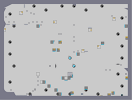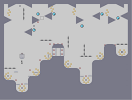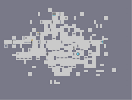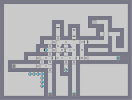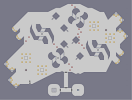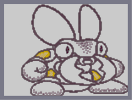From All Angles Suckiest Bunch of Sucks That Ever Sucked Life's A Glitch, Then You Die Multifarious Xyloger Bugzzy - I

## Comments

Pages: (0)

### Some

parts he did use 1/4, with thwumps. but look at the wings. Those are no snap :O

### V. Cool

This is a great N - Art.
5/5

### schweet

best shaded-N-art I've seen thats AAD (After Afternoon Dragon)

### Nice action lvl

joke.
4/5, need to colour the wings.

wings

### 4.359/5

very nice, i like this...
sorry but it rounds down to 4...

### 4.5 or 5

?
I do like it though, but I'm not a huge N-art fan

Nice job :D

### 4.5/5

Great job!
I still think Afternoon Dragon is Dragon is the best N-art...

### cool map

he's cool but i agree a red one 1 would be cool but still a 5

### Man I hated that beetle.

But you get a 5 nonetheless.

### ^_^

This should have it's place on the toprated (as it stands right now) for awhile, it deserves it.

Great aspect, near flawless detail, I love the shading.

4.5/5 (The only 5/5 on an N-Art I ever gave was on Afternoondragon Step4)

........

### Actually no,

I went over the outlines with z-snap, but the rest is c-snaped.

### awesome

d..did you use 1/4 snap for all of it? looks remarkably smooth for having any snap

### It's not amazing,

def. not bad for sure though!

3.5